Math Olympiad Test: Introduction to Euclid’s Geometry- 2

# Math Olympiad Test: Introduction to Euclid’s Geometry- 2

Test Description

## 15 Questions MCQ Test Mathematics Olympiad for Class 9 | Math Olympiad Test: Introduction to Euclid’s Geometry- 2

Math Olympiad Test: Introduction to Euclid’s Geometry- 2 for Class 9 2022 is part of Mathematics Olympiad for Class 9 preparation. The Math Olympiad Test: Introduction to Euclid’s Geometry- 2 questions and answers have been prepared according to the Class 9 exam syllabus.The Math Olympiad Test: Introduction to Euclid’s Geometry- 2 MCQs are made for Class 9 2022 Exam. Find important definitions, questions, notes, meanings, examples, exercises, MCQs and online tests for Math Olympiad Test: Introduction to Euclid’s Geometry- 2 below.
Solutions of Math Olympiad Test: Introduction to Euclid’s Geometry- 2 questions in English are available as part of our Mathematics Olympiad for Class 9 for Class 9 & Math Olympiad Test: Introduction to Euclid’s Geometry- 2 solutions in Hindi for Mathematics Olympiad for Class 9 course. Download more important topics, notes, lectures and mock test series for Class 9 Exam by signing up for free. Attempt Math Olympiad Test: Introduction to Euclid’s Geometry- 2 | 15 questions in 15 minutes | Mock test for Class 9 preparation | Free important questions MCQ to study Mathematics Olympiad for Class 9 for Class 9 Exam | Download free PDF with solutions
 1 Crore+ students have signed up on EduRev. Have you?
Math Olympiad Test: Introduction to Euclid’s Geometry- 2 - Question 1

### Which of the following statement is an axiom:

Detailed Solution for Math Olympiad Test: Introduction to Euclid’s Geometry- 2 - Question 1

The first statement is an axiom and does not require proof.

Math Olympiad Test: Introduction to Euclid’s Geometry- 2 - Question 2

### Which of the following statement is wrong?

Detailed Solution for Math Olympiad Test: Introduction to Euclid’s Geometry- 2 - Question 2

All the other statements i.e. (a) (b) and (c) are Euclid’s postulates except option (d).

Math Olympiad Test: Introduction to Euclid’s Geometry- 2 - Question 3

### The number of planes passing through three non-collinear points is:

Detailed Solution for Math Olympiad Test: Introduction to Euclid’s Geometry- 2 - Question 3

The line is formed using 3 non–collinear points.
∴ 3 non-collinear points will form 1 plane.

Math Olympiad Test: Introduction to Euclid’s Geometry- 2 - Question 4

How many lines can pass through 2 given points?

Detailed Solution for Math Olympiad Test: Introduction to Euclid’s Geometry- 2 - Question 4

Through 2 given points, 1 and only one line can be drawn.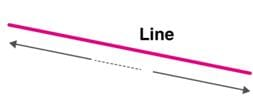Math Olympiad Test: Introduction to Euclid’s Geometry- 2 - Question 5

If 4 lines pass through a point these are said to be

Detailed Solution for Math Olympiad Test: Introduction to Euclid’s Geometry- 2 - Question 5

If three or more lines pass through the same point then the point is called point of concurrence of the given lines.

Math Olympiad Test: Introduction to Euclid’s Geometry- 2 - Question 6

Two circles are said to be congruent, if and only if:

Detailed Solution for Math Olympiad Test: Introduction to Euclid’s Geometry- 2 - Question 6

Two circles are congruent if they have equal radii.

Math Olympiad Test: Introduction to Euclid’s Geometry- 2 - Question 7

Two lines can intersect in how many points (maximum)?

Detailed Solution for Math Olympiad Test: Introduction to Euclid’s Geometry- 2 - Question 7

Two lines can intersect in one and only one point.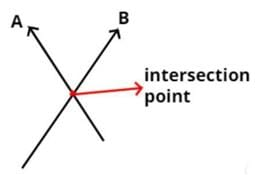Math Olympiad Test: Introduction to Euclid’s Geometry- 2 - Question 8

A point has

Detailed Solution for Math Olympiad Test: Introduction to Euclid’s Geometry- 2 - Question 8

A point has no size but we show it by a dot to know where a point is. A point has no length, no width, and has no height. A point only has its position. It means a point has no dimensions or has zero dimensions.

Math Olympiad Test: Introduction to Euclid’s Geometry- 2 - Question 9

A surface has

Detailed Solution for Math Olympiad Test: Introduction to Euclid’s Geometry- 2 - Question 9

A surface has a curved boundary and a curve contains infinite points.

Math Olympiad Test: Introduction to Euclid’s Geometry- 2 - Question 10

If an angle is such that, its complementary angle is 20°, the n angle is

Detailed Solution for Math Olympiad Test: Introduction to Euclid’s Geometry- 2 - Question 10

Let the measure of angle be x°
Then, its complementary angle will be
(90° – x°) = 20°
⇒ x = 70°

Math Olympiad Test: Introduction to Euclid’s Geometry- 2 - Question 11

The measure of an angle if the five times its complement is 12° less than twice its supplement

Detailed Solution for Math Olympiad Test: Introduction to Euclid’s Geometry- 2 - Question 11

Let the measure of angle be x° then, its complementary angle will be 90° – x° supplement = 180°– x°
∴ 5 (90° – x°) = 2 (180° – x°) – 12°
⇒ x = 34°

Math Olympiad Test: Introduction to Euclid’s Geometry- 2 - Question 12

Boundaries of surfaces and solids are:

Detailed Solution for Math Olympiad Test: Introduction to Euclid’s Geometry- 2 - Question 12

Boundary of surface is curved and boundary of solid is surface.

Math Olympiad Test: Introduction to Euclid’s Geometry- 2 - Question 13

The numbers of lines that can be drawn through 4 distinct points in a plane if none three points are collinear

Detailed Solution for Math Olympiad Test: Introduction to Euclid’s Geometry- 2 - Question 13

No. of lines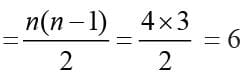Math Olympiad Test: Introduction to Euclid’s Geometry- 2 - Question 14

In problem – 24 number of lines that can be drawn if 3 of the 4 points are collinear will be

Detailed Solution for Math Olympiad Test: Introduction to Euclid’s Geometry- 2 - Question 14

No. of lines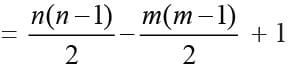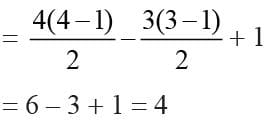Math Olympiad Test: Introduction to Euclid’s Geometry- 2 - Question 15

Every line segment has:

Detailed Solution for Math Olympiad Test: Introduction to Euclid’s Geometry- 2 - Question 15

Every line segment has one and only one midpoint.

## Mathematics Olympiad for Class 9

1 videos|43 tests
 Use Code STAYHOME200 and get INR 200 additional OFF Use Coupon Code
Information about Math Olympiad Test: Introduction to Euclid’s Geometry- 2 Page
In this test you can find the Exam questions for Math Olympiad Test: Introduction to Euclid’s Geometry- 2 solved & explained in the simplest way possible. Besides giving Questions and answers for Math Olympiad Test: Introduction to Euclid’s Geometry- 2, EduRev gives you an ample number of Online tests for practice

## Mathematics Olympiad for Class 9

1 videos|43 tests